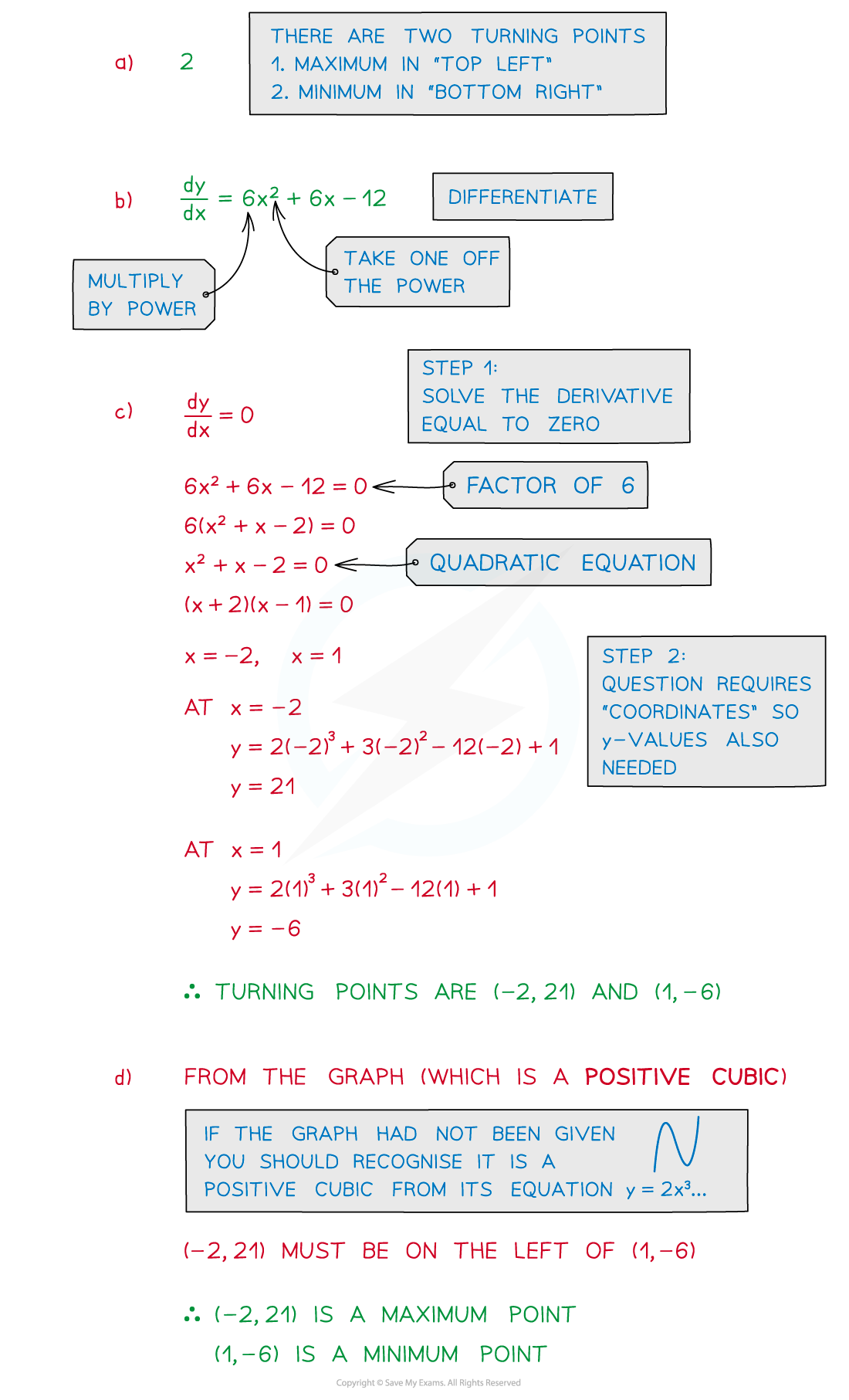# Edexcel IGCSE Maths 复习笔记 3.11.2 Differentiation - Turning Points

Edexcel IGCSE Maths 复习笔记 3.11.2 Differentiation - Turning Points

#### Remind me of the rule for differentiating powers

• If y = kxn then dy/dx = knxn-1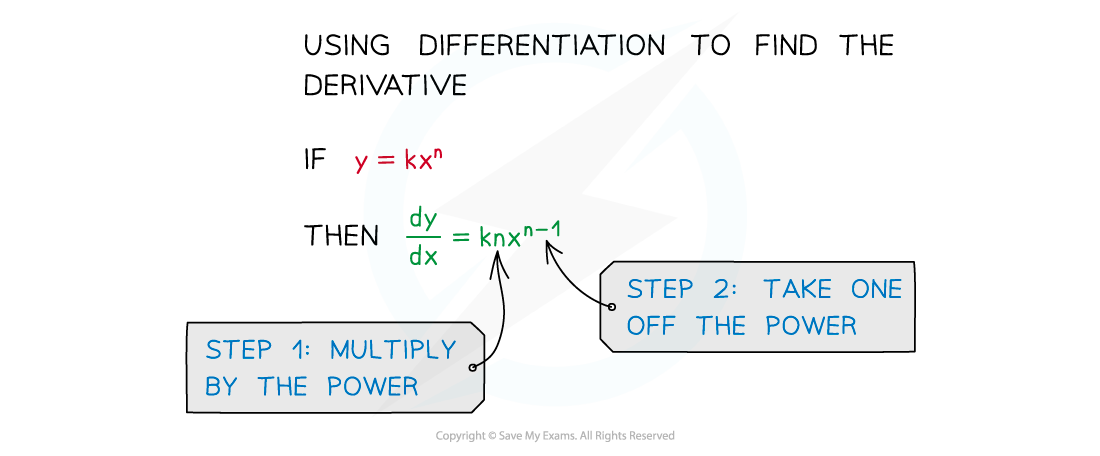#### What is a turning point?

• The easiest way to think of a turning point is that it is a point at which a curve changes from moving upwards to moving downwards, or vice versa
• Turning points are also called stationary points
• Ensure you are familiar with Differentiation – Basics before moving on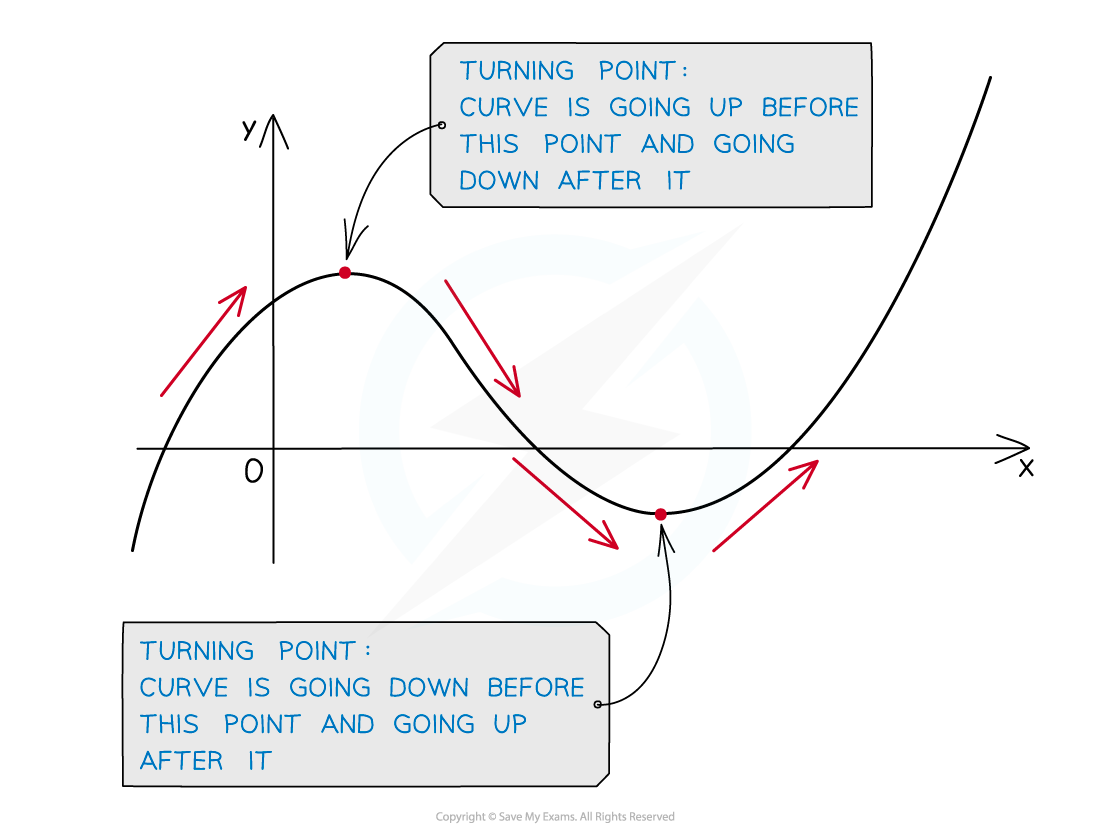• At a turning point the gradient of the curve is zero
• If a tangent is drawn at a turning point it will be a horizontal line
• Horizontal lines have a gradient of zero

• This means at a turning point the derivative or gradient function equals zero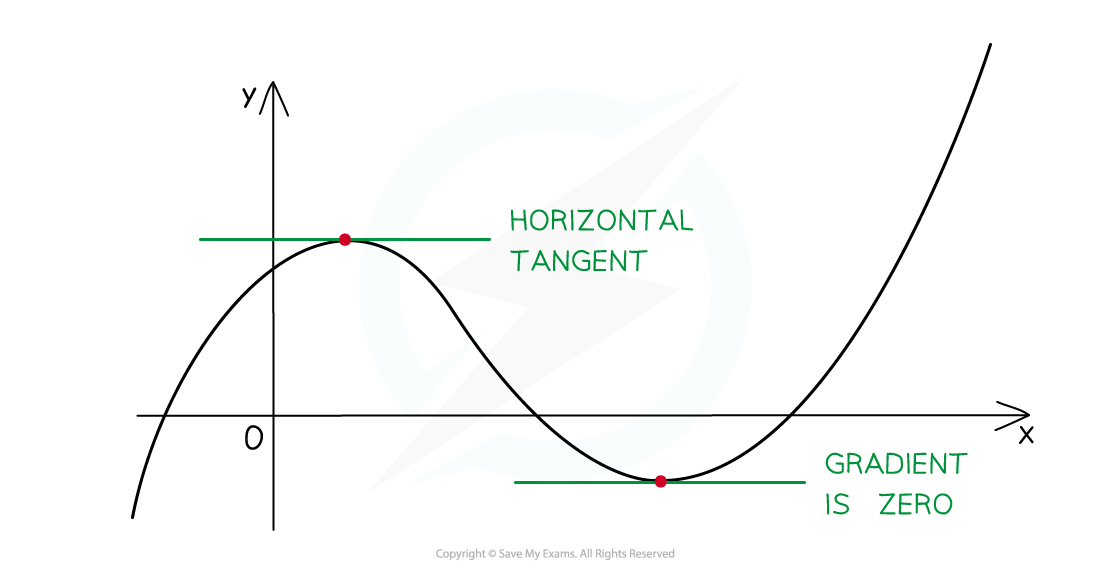#### How do I know if a curve has turning points?

• You can see from the shape of a curve whether it has turning points or not
• At IGCSE, two types of turning point are considered:
• Maximum points – this is where the graph reaches a “peak
• Minimum points – this is where the graph reaches a “trough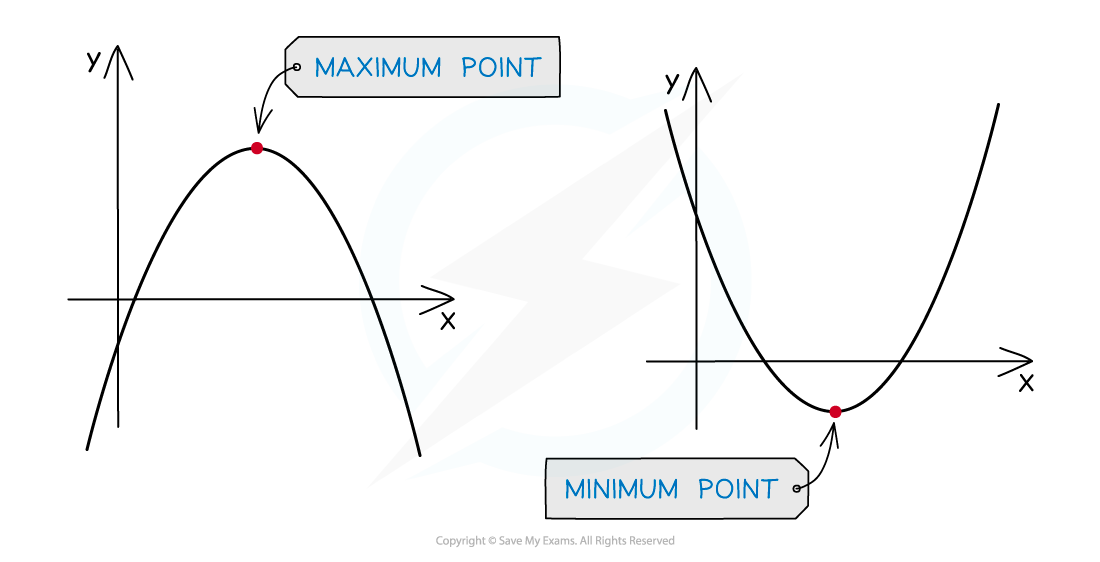• These are sometimes called local maximum/minimum points as other parts of the graph may still reach higher/lower values

#### How do I find the coordinates of a turning point?

• STEP 1  Solve the equation of the gradient function (derivative) equal to zeroie. solve dy/dx = 0

This will find the x-coordinate of the turning point

• STEP 2  To find the y-coordinate substitute the x-coordinate into the equation of the graph

ie. substitute x into “y = ...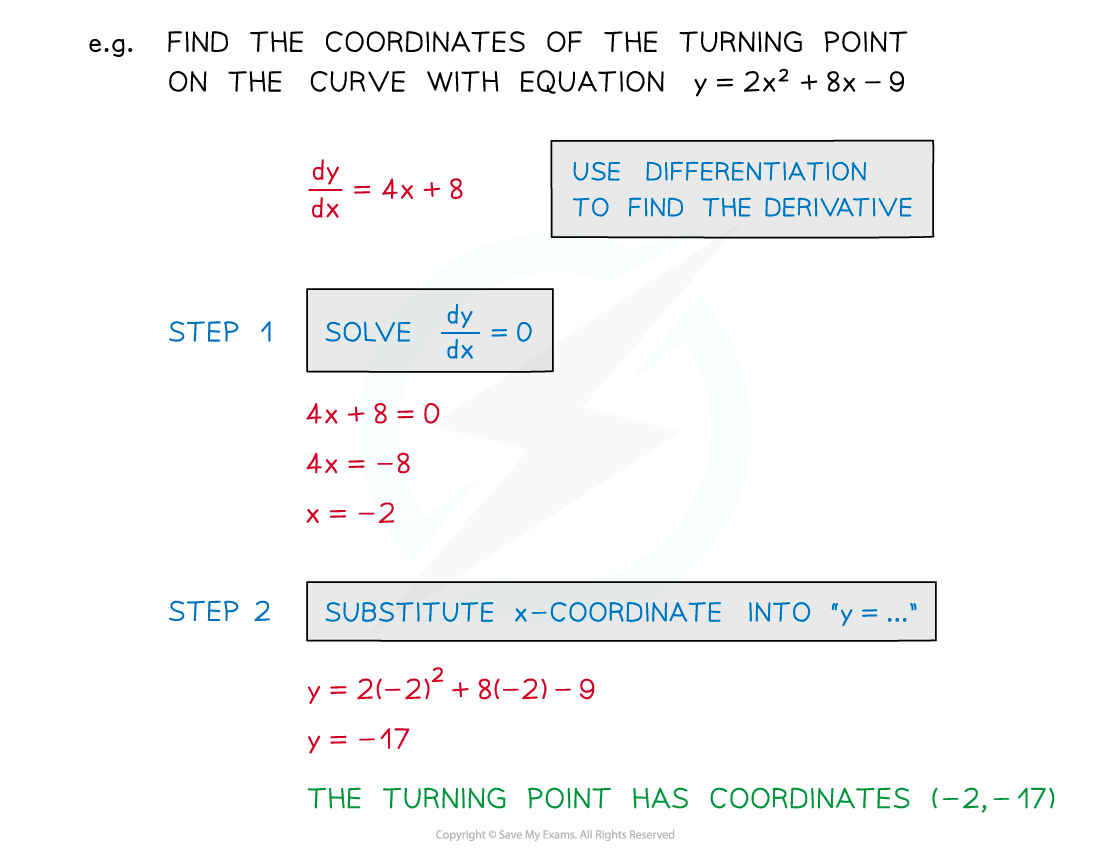#### How do I know which point is a maximum and which is a minimum?

• The easiest way to do this is to recognise the shape of the curve
• ... either from a given sketch of the curve
• ... a sketch of the curve you can quickly draw yourself

(You may even be asked to do this as part of a question)

• ... the equation of the curve

• For parabolas (quadratics) it should be obvious ...
• ... a positive parabola (positive x2 term) has a minimum point
• ... a negative parabola (negative x2 term) has a maximum point• Cubic graphs are also easily recognisable ...
• ... a positive cubic has a maximum point on the leftminimum on the right
• ... a negative cubic has a minimum on the leftmaximum on the right#### Exam Tip

Read questions carefully – sometimes only the x-coordinate of a turning point is required.Differentiating accurately is crucial in leading to equations you can work with and solve.

#### Worked Example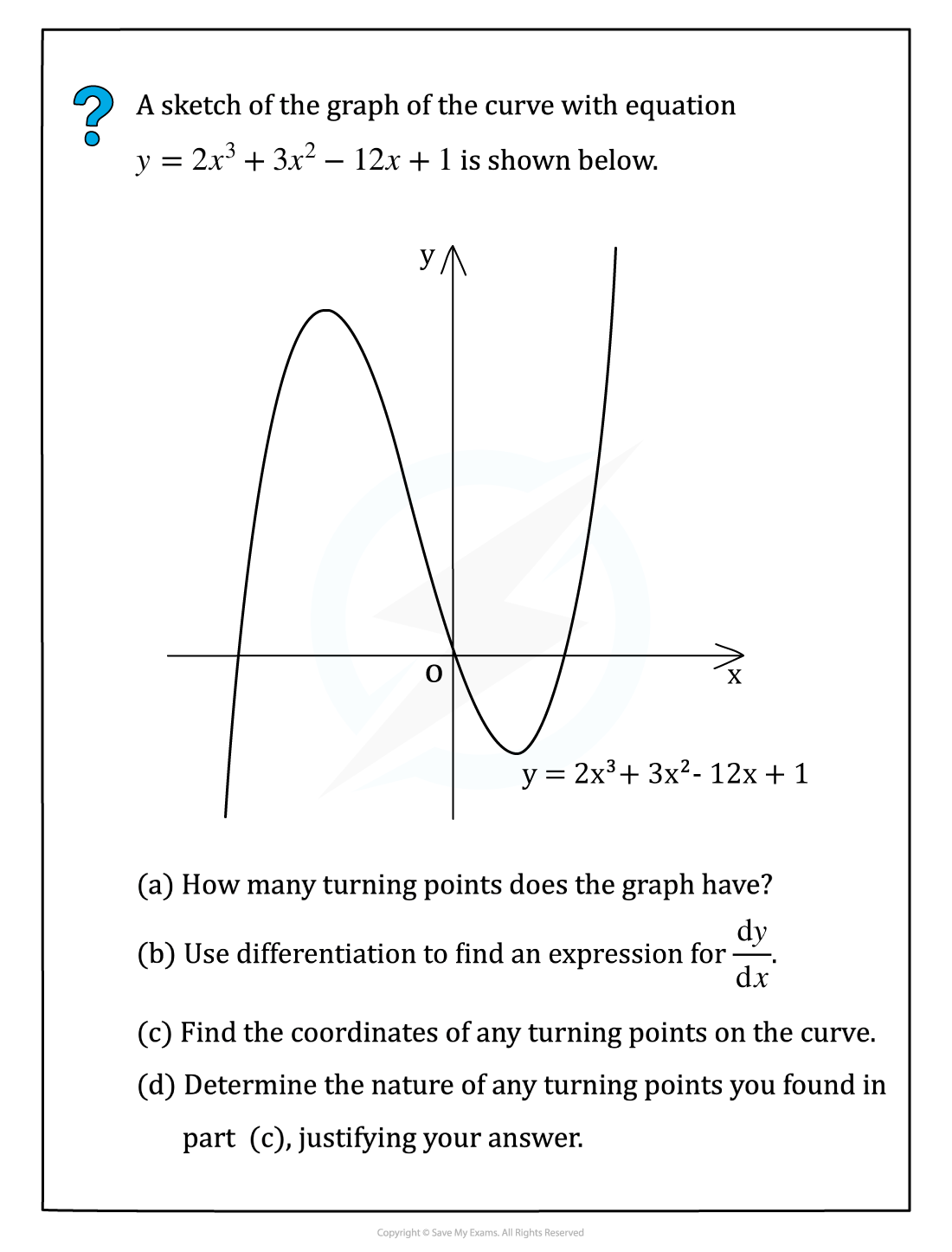#### Worked Example